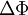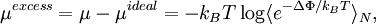2022: SklogWiki celebrates 15 years on-line

# Widom test-particle methodThis article is a 'stub' page, it has no, or next to no, content. It is here at the moment to help form part of the structure of SklogWiki. If you add sufficient material to this article then please remove the {{Stub-general}} template from this page.
The Widom test-particle method, proposed by Benjamin Widom , is an elegant, general simulation technique to obtain the excess chemical potential of a system. A so-called test particle is introduced in a random location, and$\Delta\Phi$, the difference in internal energy before and after the insertion, is computed. For pairwise interactions, this would become be the interaction potential energy between the randomly placed test particle and the$N$ particles that the system is comprised of. The particle is not actually inserted, at variance with grand canonical Monte Carlo.$\mu^{excess} = \mu -\mu^{ideal} = -k_BT \log \langle e^{-\Delta\Phi/k_BT}\rangle_N ,$
where$k_B$ is the Boltzmann constant and$T$ is the temperature.